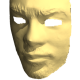# Correct barrel distortion without reference images

Hello,

I want to correct a simple barrel distortion whithout using calibration images. In imagemagick-convert I just call e.g. convert img -distort Barrel "0.0 0.0 -0.1 1.0". This is sufficient for my camera. In OpenCV it seems to be much more complicated than this.

Is there any easy way comparable to imagemagick to do this simple distortion correction? I just want to avoid calling imagemagick from my python script since OpenCV should be able to do the entire job.

edit retag close merge delete

Sort by » oldest newest most votedAs usual, there are benefits and drawbacks for using OpenCV for a certain task. This comment is trivially true for basically any tool at your disposal. If ImageMagick is a much easier route for your purpose, then by all means use the best handy tool you have.

Step 1. Generate two matrices which contain just the coordinates of each pixel. This is same as the MATLAB command meshgrid (link). The equivalent steps in OpenCV are found in this answer.

Step 2. Using the matrices of x and y values, convert them into a matrix of "r", which is the distance from each pixel to the center of the image.

Step 3. Likewise, compute the value of "theta" from x and y. This "theta" is necessary for Step 6.

Step 2 and 3 together forms the basics of "Cartesian to Polar coordinate conversion".

Step 4. Computer the matrices of "r" raised to the second and third power.

Step 5. Apply the formula seen in the ImageMagick documentation on the polynomial of "r". Let the new result to be "r prime".

Step 6. With the "r prime" from Step 5, and the "theta" from Step 3, convert the polar coordinates back to Cartesian coordinates, "x prime" and "y prime"

Step 7. "x prime" and "y prime" can be used with remap to generate the final result.

Caveat 1.

When applying the steps above, it is very important to distinguish whether one is performing the "forward mapping" or the "inverse mapping". Inverse mapping is closer to the everyday programming concept of "array lookup" - given the output array index, look up the output's value from the input at the input array index.

Caveat 2.

For typical sizes of photographic images (about several thousand pixels across in each dimension), the value of "r" will be roughly similar. When such values are evaluated in a cubic polynomial, if the signs of some polynomial coefficients are opposite, it is possible that catastrophic cancellation can occur, if the 32-bit floating point (CV_32F) is used. MATLAB users might pay less attention from this problem because MATLAB defaults to using 64-bit floating point always. Working around this issue is beyond the scope of this question. I encourage any advanced OpenCV users to share your opinions on this issue.

(My memory is too weak to recall whether Horner's polynomial evaluation can mitigate this issue.)

Caveat 3.

The approach above will use lots of memory, as one might suspect. C or C++ programmers would tend to write a two-level for-loop, and calculate the "x prime" and "y prime" from the equations given the loop variables "x" and "y". That way only two coordinate matrices would ever be allocated. Users of Python would probably look for something like numpy.fromfunction (documentation), such as this example. (Disclaimer: since I do not use Python, I do not know the performance or memory characteristics of this function.)

I will happily let any ...

moreBarrel distortion removal can be considered as remapping a given pixel (x,y) from the original image f into a image g, by a function h(x,y), where the mapping function is the one used by ImageMagick (which you can see here ).

In OpenCV, there is the function cv::remap where you give two mapping images for the x and y coordinates, with the desired interpolating function (nearest neighbour, bilinear, etc.). There is a pretty good tutorial in the OpenCV documentation here showing how you can generate custom maps for remapping of images. Instead translation/rotation in the example you should use the ImageMagick equations, with the parameters that you use.

I hope that this helps.

more

So your suggestion is to build my own mapping and provide this to remap function? This seems to be quite slow in execution (in Python). That's the reason I wanted to apply a "real" OpenCV function.

The "real" OpenCV function is the cv::remap function. All that you have to compute the mapping matrices only once, as initialization, and give it as parameter to the remap function. It will un-distort the frame using any optimization options available in OpenCV. If you read the documentation, you will see that "cv::undistort", for lens distortion removal, uses "remap" internally.

Yes, I'm sure You are right but I don't really get it. Using only one main parameter (with imagemagick) is much simpler and doesn't need any deeper understanding of transformation matrices. Maybe you have some code snippets (in Python)? Thanks in advance.

Official site

GitHub

Wiki

Documentation